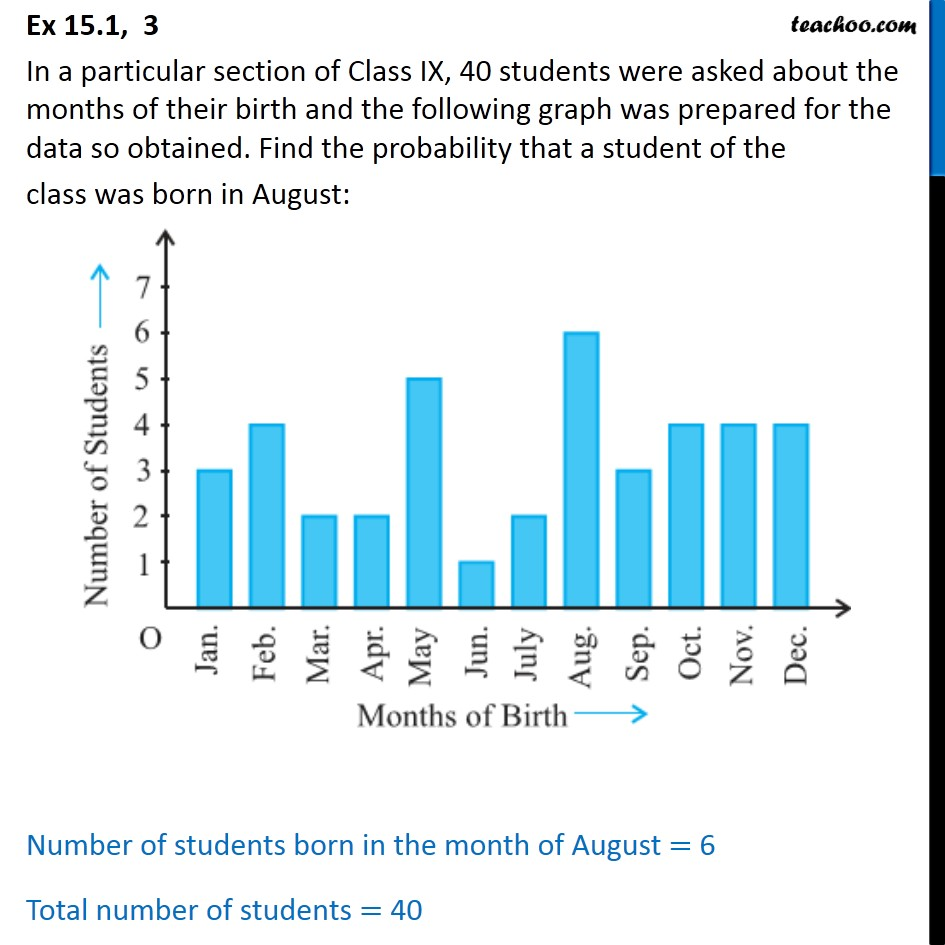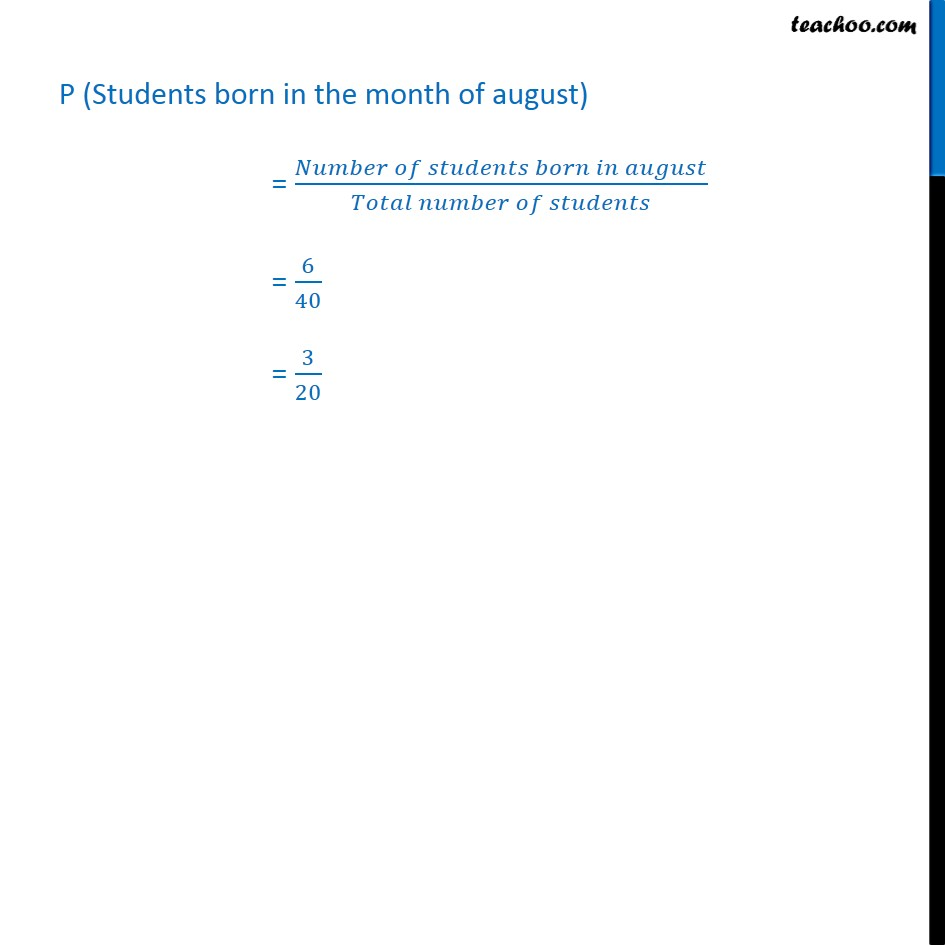1. Chapter 15 Class 9 Probability (Term 2)
2. Serial order wise
3. Ex 15.1

Transcript

Ex 15.1, 3 In a particular section of Class IX, 40 students were asked about the months of their birth and the following graph was prepared for the data so obtained. Find the probability that a student of the class was born in August: Number of students born in the month of August = 6 Total number of students = 40 P (Students born in the month of august) = (𝑁𝑢𝑚𝑏𝑒𝑟 𝑜𝑓 𝑠𝑡𝑢𝑑𝑒𝑛𝑡𝑠 𝑏𝑜𝑟𝑛 𝑖𝑛 𝑎𝑢𝑔𝑢𝑠𝑡)/(𝑇𝑜𝑡𝑎𝑙 𝑛𝑢𝑚𝑏𝑒𝑟 𝑜𝑓 𝑠𝑡𝑢𝑑𝑒𝑛𝑡𝑠) = 6/40 = 3/20

Ex 15.1